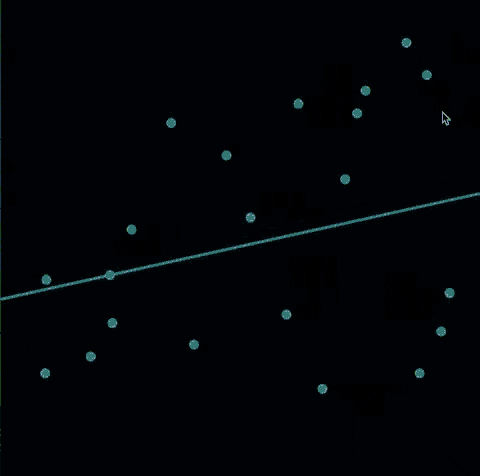# Hands-on Linear Regression Using Sklearn!Linear regression in Machine Learning is a kind of analysis where there are both dependent and independent variables. Their relationship is depicted here by a linear line from the provided data. This technique is mainly used for predicting the results.

The data and the values from the linear regression are used to find the Mean Squared Error (MSE) to change the values of the variables so that they have the least value possible and get the most accurate results.

While there are several tools that are usually used for linear regression Sklearn is one of the popular tools.

What is Sklearn?

Sklearn is the short form for Scikit Learn which in Python Language, an open-source ML library to help unify the various tools of algorithms in machine learning.Sklearn precisely works as a one-stop solution that helps with importing, preprocessing, plotting, and predicting data.

Its versatility, powerful nature, ample documentation, and active nature make it one of the popular toolkits in ML.

Linear Regression in machine learning

Linear regression comes at the last stage of the ML processing. Though there are several tools for the same, Sklearn is used more because of its versatility. When there is a log of consistent data it is easier to predict future patterns. The various steps towards the implementation of linear regression are,

• Training the model results in dividing the data into dependent and independent variables.
• Later it is split the data into train and test sets.
• It is the test set that will be used for the predictions.
• Explore the results to find the suitability of the data for linear regression. A low accuracy data shows the non-compatibility of data to this.
• Narrow down the data to a smaller portion and repeat the same steps above.
• Now the data should be able to show the MSE which can be used for better predictions.

Importance of Linear regression

Linear regression is important as it determines the accuracy of the prediction from the data given and also finds out the predictors that are significant for better results.It is useful in determining the power between the dependent and independent variables such as sales and expenditure, or in the case of health care dose and its effects on the body, etc.

Analyzing the regression model explains how much the variables do change with respect to each other. Forecasting weather or its impact and changes is a fine example of this application. In the case of sales and marketing, it explains how much increase is possible in income with a specific amount of expenditure.

Finally, it is one of the main tools for predicting future trends and possibilities. It can be used for a future prediction of any time span. Linear regression using Sklearn provides a near-accurate prediction of the values.

In addition, there are different types of linear regression - simple or multiple linear regression. Each type is suitable for a different purpose or type of data available.

Conclusion

Its possibility is too vast that it requires proper Linear Regression Training to use the best data for getting the most accurate output. Once learned it is easier to predict data and values in the fields of sales, marketing, financial assessments, insights into consumer behavior, risks in finance or loans, etc.

As already mentioned, the accuracy of the prediction highly depends on how compatible the data is for the linear regression model. The Sklearn method is a far better option to determine the same and can provide the closest values to help with a better prediction model for the business.

## drop us a message and keep in touch